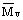(1) The numerical value of cosmic parameter

The results computed by using formulae of the outmost layer are listed in Table 2. Whereis value in agreement with optimal fitting curve.  Several restrictions have been advanced to lessen limits of selection.

1)      There is  MVT = 7-10ML  at galactic center, therefore

1.4  < ρ <  2.0

2)      a < 4 [C.Misner 1973];  rs > 4.

3)      Maximum value of Hubble constant is 95 [Van den Bergh], it coincide with  Hmax  in this theory, then

H < 77

4)      As redshift distance reduced, we have< 20.3

5)      Suppose  Zmax > 5

Thus we obtain Table 3. It can be see from Table 2 and Table 3 that the reasonable limit is

H = 65 - 70               M = 0.9 - 1.2

a = 3.5 - 3.7              ρ = 1.6 - 2.0

We may chose some group of parameter according to the viewpoint of self. The group which I have picked out is

H = 68             ρ = 1.75             M = 1.0

a = 3.59            μ = 0.402

The results computing other parameter were written in Table 1.